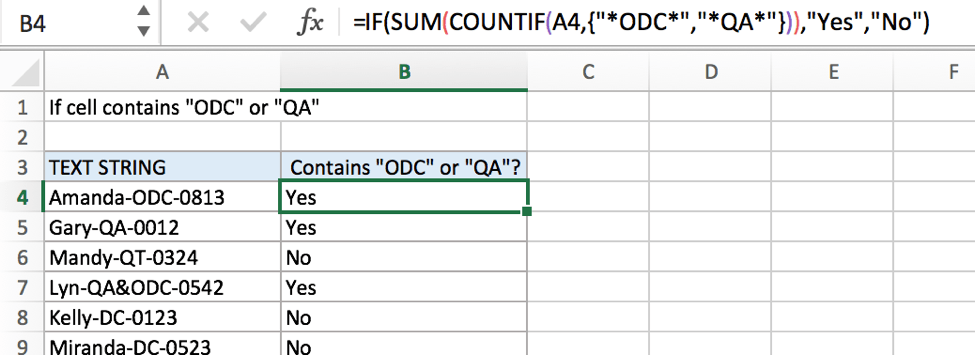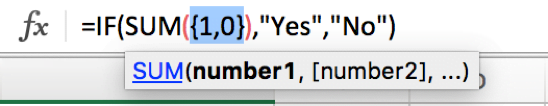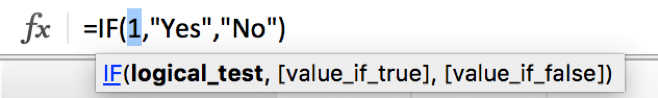# If Cell Contains Either Text1 or Text2

IF function is frequently used in Excel worksheet to return “true value” or “false value” based on the logical test result. If you want to see if cell contains certain substring1 like “abc” or substring2 like “def”, and returns true of false based on test result, you can build a formula based on IF function and together with SUM function and COUNTIF function.FORMULA

To test if cell contains certain text1 or text2, the generic formula is:

=IF(SUM(COUNTIF(A1,{“*Text1*”,”*Text2*”})),”true value”,”false value”)

Formula in this example

=IF(SUM(COUNTIF(A4,{“*ODC*”,”*QA*”})),”Yes”,”No”)

In this example, we want to know the result of “if cell contains “ODC” or “QA””, the result is either “Yes” or “No”, Excel IF function can handle this case properly. And to test if cell contains certain substring, we can use COUNTIF function.

EXPLANATION

In this example, we want to know if cells in A column contain either “ODC” or “QA”, so we use COUNTIF function to see if they are included in text string. If yes, a positive number is retuned, if none of them is found in the test string, zero is returned. COUNTIF is the core in the formula =IF(SUM(COUNTIF(A4,{“*ODC*”,”*QA*”})),”Yes”,”No”) actually.

For example, to test if A4 contains “ODC” or “QA”, we can run formula COUNTIF(A4,{“*ODC*”,”*QA*”}) to see if A4 contains the supplied substrings.

In this formula, as we supply two substrings “ODC” and “QA”, so we create array constant {“*ODC*”,”*QA”} to show the collection of supplied substrings.

• Asterisk (*) is a wildcard, it stands for one or more characters, if we remove “*” from substrings like {“ODC”,”QA”}, our expectation “cell contains “ODC” or “QA”” is changed to “cell equals “ODC” or “QA””.
• As the two substrings are given in precondition, so they are hard-coded, we use comma to separate them, you can enter your substrings in you case.
• “*ODC*”, “*QA*” should be quoted by double quotes, if missing double quotes #NAME? error displays instead and it signifies some errors should be corrected in this formula.
• The array constant is started and ended with curly brace “{}”.

When running the formula, COUNTIF function compares text string in cell with the items in array constant one by one. In this COUNTIF, there is only one cell A4, text string “Amanda-ODC-0813” only contains the first substring “ODC”, thus COUNTIF(A4,{“*ODC*”,”*QA*”}) returns “1” for “ODC”, and returns “0” for “QA” after comparing with “Amanda-ODC-0813”, results are saved in array {1,0} and delivered to SUM function.SUM is within IF function as its argument “logical_test”. SUM function add up all items in array {1,0} and delivery result to IF function directly.Logical test result is a positive number, so IF function returns true value “Yes” to B4.

COMMENT

• COUNTIF function supports wildcards like “?” and “*”.
• COUNTIF function is case-insensitive.

### Related Functions

• Excel COUNTIF function
The Excel COUNTIF function will count the number of cells in a range that meet a given criteria. This function can be used to count the different kinds of cells with number, date, text values, blank, non-blanks, or containing specific characters.etc.= COUNTIF (range, criteria)…
• Excel IF function
The Excel IF function perform a logical test to return one value if the condition is TRUE and return another value if the condition is FALSE. The IF function is a build-in function in Microsoft Excel and it is categorized as a Logical Function.The syntax of the IF function is as below:= IF (condition, [true_value], [false_value])….
• Excel SUM function
The Excel SUM function will adds all numbers in a range of cells and returns the sum of these values. You can add individual values, cell references or ranges in excel.The syntax of the SUM function is as below:= SUM(number1,[number2],…)…